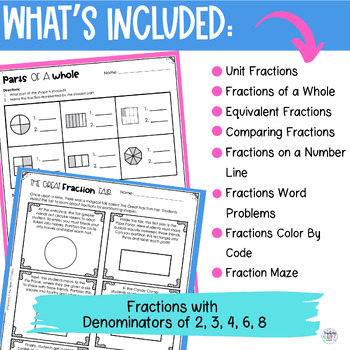# Fractions Worksheet | Comparing Fractions, Equivalent Fractions

Rated 4.86 out of 5, based on 135 reviews
135 Ratings;
3rd - 4th, Homeschool
Subjects
Resource Type
Standards
Formats Included
• PDF
Pages
26 pages
Report this resource to TPT
##### Also included in
1. These Rounding, Multiplication, Division, Fractions and Place Value worksheets are just what you need for your 3rd-grade classroom! This big bundle of Quick Print &amp; Go printable worksheets is perfect for math lessons, math centers, or homework. If you often need to reteach these important math c
Price \$26.20Original Price \$32.75Save \$6.55
2. Get your 3rd-grade students excited about fractions with this bundle of fun and challenging fractions activities! From whole-class activities to small group interventions, from math stations to homework activities - it's all covered. And the best part? You'll save loads of time prepping for your fra
Price \$19.20Original Price \$24.00Save \$4.80

### Description

These differentiated fraction worksheets will solve the problem of finding just the right fraction activity for all your students! Students will practice fractions using bar models, number lines, and fraction shapes to learn about unit fractions, parts of a whole, fractions on number lines, comparing fractions, and equivalent fractions.

PLEASE SEE PREVIEW FOR A CLOSER LOOK!

Save 20% on this 3rd Grade Math Worksheets Bundle!

Included in this FRACTIONS ACTIVITY packet:

♦ Unit Fractions

♦ Parts of a Whole

♦ Fractions on a Number Line

♦ Comparing Fractions with Like Numerators

♦ Comparing Fractions with Like Denominators

♦ Equivalent Fractions

♦ Create Fraction Designs

♦ Given This Number Line

♦ Solve Fraction Problems

♦ Why Are These Fractions Equal?

♦ Given This Rectangle

♦ Find a Fraction That Is…

♦ How to Use Suggestions

Skills practiced with these fraction printables:

• Create and write fraction names

• Partition and name fractions

• Use number lines to plot and compare fractions

• Find equivalent fractions using bar models

• Solve fraction word problems using models

• Reason and explain fractions on number lines

• Compare fraction sizes

• Write to explain fraction equivalence and number line placement

──────────────────────────

These worksheets are perfect for many different uses:

• Whole Group Instruction/Review

• Formative Assessments

• Independent Practice

• Partners

• Centers

• Remediation

──────────────────────────
Common Core Math Standards Addressed in this Bundle:

NF.A.1- Understand a fraction 1/b as the quantity formed by 1 part when a whole is partitioned into b equal parts; understand a fractiona/b as the quantity formed by a parts of size 1/b.

NF.A.2- Understand a fraction as a number on the number line; represent fractions on a number line diagram.

NF.A.3- Explain equivalence of fractions in special cases, and compare fractions by reasoning about their size.

──────────────────────────

More math worksheet resources in my store:
Rounding to the Nearest 10 and 100 Worksheets - Print & Digital

"Don't forget to leave feedback -you will receive TPT credits that you can use toward future purchases!

Thank you for visiting Teaching in the Heart of Florida!

Total Pages
26 pages
Included
Teaching Duration
Other
Report this resource to TPT
Reported resources will be reviewed by our team. Report this resource to let us know if this resource violates TPT’s content guidelines.

### Standards

to see state-specific standards (only available in the US).
Understand a fraction 1/𝘣 as the quantity formed by 1 part when a whole is partitioned into 𝘣 equal parts; understand a fraction 𝘢/𝑏 as the quantity formed by 𝘢 parts of size 1/𝘣.
Understand a fraction as a number on the number line; represent fractions on a number line diagram.
Explain equivalence of fractions in special cases, and compare fractions by reasoning about their size.